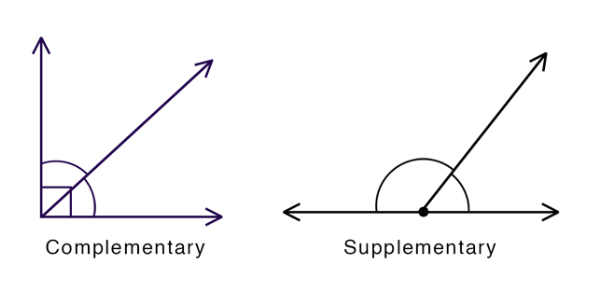# Complementary And Supplementary Angles Quiz

10 Questions | Total Attempts: 5188SettingsUse your knowledge for this Complementary and supplementary angles quiz to answer the questions. YOU DO NOT NEED TO PUT THE DEGREE SYMBOL AS PART OF YOUR ANSWER. MAKE SURE THAT YOU DO NOT PRESS THE SPACEBAR BEFORE ENTERING YOUR ANSWER, AND DO NOT PRESS THE SPACEBAR BETWEEN ANSWERS THAT HAVE MORE THAN ONE DIGIT.

• 1.
These two angles in the image are the examples of ________ angles.
• 2.
Find the missing angle.
• 3.
Find the missing angle.
• 4.
Find the missing angle.
• 5.
Find the missing angle.
• 6.
Find the missing angle.
• 7.
Find the missing angle.
• 8.
If the smaller angle in the image is 30 degrees, then the bigger one is _____ degrees.
• 9.
Find the missing angle.
• 10.
Find the missing angle.
Related TopicsBack to top